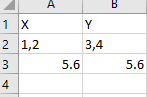# Check decimal or whole numberHow to check whether number are whole or decimal if it is whole it should multiply (1,2)(3,4)
If it is decimal it should multiply 5.6
5.6

Also is this condition correct to check?Hi,

I didnt get the above sentence, however the following will check what you expect.

Condition for checking decimal

`````` Double.TryParse(CurrentRow("X").ToString,System.Globalization.NumberStyles.AllowDecimalPoint,System.Globalization.CultureInfo.InvariantCulture,New Double) AndAlso Double.TryParse(CurrentRow("Y").ToString,System.Globalization.NumberStyles.AllowDecimalPoint,System.Globalization.CultureInfo.InvariantCulture,New Double)
``````

Condition for comma separated value

``````System.Text.RegularExpressions.Regex.IsMatch(CurrentRow("X").ToString,"^\d+,\d+\$") AndAlso System.Text.RegularExpressions.Regex.IsMatch(CurrentRow("Y").ToString,"^\d+,\d+\$")
``````

Sample20230110-5L.zip (8.9 KB)

Regards,

what i am trying to do is if number is (1,2) and (1,3) then output should be (13)+(24)
if number are ((5.6),(1.2)) and ((2.3),(2.4)) then output should be (5.6)*(2.3)+(1.2)+(2.4)
if number are 1 and 2 normal multiplications
Match collection while help?/